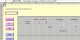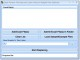Quadratic Formula Helper 0.1 Quadratic Formula Helper is a small application for quickly finding the value(s) of X from a quadratic formula. FreewareDynamic Formula 1.0 Dynamic Formula is a RbScript subclass which can be used to allow end users to enter in their own variables and values which you, the developer, can use as a dynamic formula which is embedded into your code. FreewareQuadratic Formula 1.1 Quadratic Formula is a simple program that will solve the Quadratic Formula for you. FreewareFractographer 2.0.3 Fractographer is a program to plot the fractals of mathematical functions. FreewareStupor 1.0 Stupor is based on a simple formula. FreewareFormula Broomstick 1.3 Broom race simulator, 17 tracks, a legend based upon Harry Potter adventures SharewareExcel Absolute Relative Reference Change Software 7.0 Change formula references from absolute to relative or relative to absolute. SharewareCalcService 3.2 This service calculates the result of a selected formula within all Cocoa-applications (e. FreewareSpreadsheet Composer 2.0 An Excel add-in for automatic construction and management of complex formula. FreewareExcel Remove Formulas and Leave Value In Multiple 7.0 Replace formulas with the visible value of the cell. Shareware